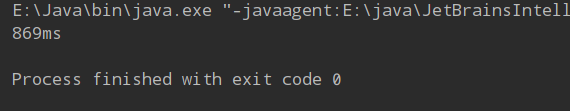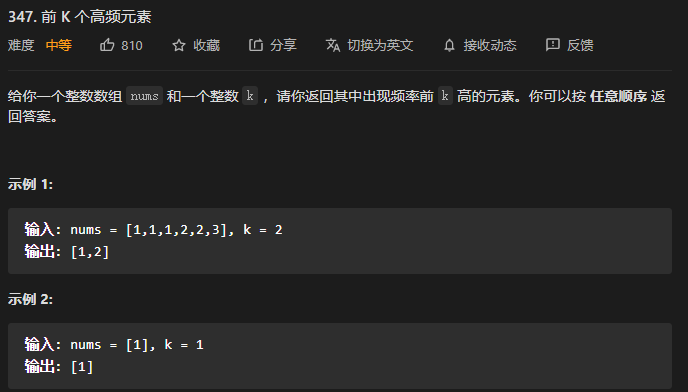• topk问题 小顶堆
2021-05-08 15:22:02
package com.heu.wsq.niuke.top200;

import java.util.ArrayList;
import java.util.Comparator;
import java.util.PriorityQueue;

/**
* TOP K问题
* @author wsq
* @date 2021/5/8
* 题目描述
* 给定一个数组，找出其中最小的K个数。例如数组元素是4,5,1,6,2,7,3,8这8个数字，则最小的4个数字是1,2,3,4。如果K>数组的长度，那么返回一个空的数组
*
* 示例1
* 输入：
*      [4,5,1,6,2,7,3,8],4
* 输出：
*      [1,2,3,4]
*/
public class GetLeastNumbers {
/**
* 大顶堆
* 使用大顶堆保存k个值，下一次新来的值v需要跟堆顶比较，
* 如果堆顶元素大于v，则将新此时的堆顶弹出，将v加入到大顶堆中
* 如果堆顶元素小于v，则直接抛弃v
* @param input
* @param k
* @return
*/
public ArrayList<Integer> GetLeastNumbers_Solution(int [] input, int k) {
if(input == null || input.length < k){
return new ArrayList<Integer>();
}
PriorityQueue<Integer> pq = new PriorityQueue<Integer>(new Comparator<Integer>(){
public int compare(Integer o1, Integer o2){
return o2 - o1;
}
});
int size = 0;
for(int i = 0; i < input.length; i++){
pq.offer(input[i]);
size++;
if(size > k){
pq.poll();
}
}
ArrayList<Integer> ans = new ArrayList<>();
while(!pq.isEmpty()){
}
return ans;
}

/**
* 快速排序的分治思想
* 通过partition将数组分为两部分，
* 索引p之前：数值小于索引p对应的元素值
* 索引p之后：数值大于索引p对应的元素值
* 如果p+1正好为k个元素，则索引p就是要寻找的位置，p之前的正好k个元素
* @param input
* @param k
* @return
*/
public ArrayList<Integer> GetLeastNumbers_Solution2(int [] input, int k) {
if (input == null || input.length < k || k==0){
return new ArrayList<>();
}
int left = 0;
int right = input.length - 1;
int p = 0;
while(left <= right){
p = partition(input, left, right);
if(p + 1 == k){
break;
}else if(p + 1 > k){
right = p - 1;
}else if(p + 1 < k){
left = p + 1;
}
}
ArrayList<Integer> ans = new ArrayList<>();
for(int i = 0; i <= p; i++){
}
return ans;
}

private int partition(int[] input, int left, int right) {
int v = input[left];
int i = left + 1;
int j = right;
while(true){
while(i <= right && input[i] <= v){
i++;
}
while(j >= left && input[j] > v){
j--;
}
if(i >= j){
break;
}
swap(input, i, j);
}
swap(input, left, j);
return j;
}

private void swap(int[] input, int i, int j) {
int tmp = input[i];
input[i] = input[j];
input[j] = tmp;
}
}java 数据结构
更多相关内容
• 首先我们需要构建一个小顶堆 我们可以用PriorityQueue这个优先队列,它给我们从小到大排序好了的,至于什么是小顶堆可以去看看堆和数的概念. PriorityQueue pq = new PriorityQueue<>(); 我们需要对小顶堆的堆...

## 首先我们需要构建一个小顶堆

我们可以用PriorityQueue这个优先队列,它给我们从小到大排序好了的,至于什么是小顶堆可以去看看堆和数的概念.

PriorityQueue pq = new PriorityQueue<>();

## 我们需要对小顶堆的堆顶与插入的数进行比较

private int k = 1000;  //从文件中找到最大的一千个数
PriorityQueue<Integer> pq = new PriorityQueue<>(k);
public void seclet(int num){
if (pq.size() < k){  //往堆中添加文件的前面一千个数
}else if (pq.peek() < num){  //如果堆顶小于文件中传递的数
pq.poll();    //删除堆顶
}
}


## 获取文件中的数,并进行判断

public void run(){
try {
String len;
while ((len = br.readLine())!=null){
seclet(Integer.parseInt(len));
if (pq.peek() == 9999){   //这个if判断看情况,这里我已知小顶堆中一千个最大的都是9999,为了效率问题,如果没有要求可以不用写
break;
}
}
BufferedWriter bw = new BufferedWriter(new FileWriter("n.txt"));
for (int i = 0; i < k && !pq.isEmpty(); i++) {
bw.write(pq.poll().toString());   //将文字以字符串的格式传进文件,否则会乱码
bw.write("\n");
}
br.close();
bw.close();
} catch (Exception e) {
e.printStackTrace();
}
}


## 最后看看从10241024128个随机数中取出1000个最大的数并写入文件中一共花费多少时间

public static void main(String[] args) {
long start = System.currentTimeMillis();
T2 t2 = new T2();
t2.run();
System.out.println(System.currentTimeMillis()-start + "ms");
}


运行结果:可以看出来是非常快速的
怎么生成一亿个随机数快速的写入文件中可以看看前面的一篇文章
有什么问题我非常乐意帮忙解决,因为我也是小白,想要磨炼磨炼

展开全文java 堆排序
• 我们在小顶堆里面存储够k个元素对应的频次即可； class mycomparison{ public: bool operator() ( pair<int,int>& lhs,pair<int,int>& rhs) { return lhs.second>rhs.second;//小顶堆，...解题思路：小顶堆顶部存储的是较小的值，从下到达排序的叫小顶堆；我们在小顶堆里面存储够k个元素对应的频次即可；

class mycomparison{
public:
bool operator() ( pair<int,int>& lhs,pair<int,int>& rhs)
{
return lhs.second>rhs.second;//小顶堆，这里要注意，小顶堆的定义方式是">"
}
};//定义一个仿函数来自定义小顶堆的排序顺序
class Solution {
public:

vector<int> topKFrequent(vector<int>& nums, int k) {
unordered_map<int,int> mp;
vector<int> ans(k,0);
for(int& num:nums)
{
mp[num]++;
}
//自定义一个小顶堆；
priority_queue<pair<int,int>,vector<pair<int,int>>,mycomparison> pri_que;
for(unordered_map<int,int>::iterator it=mp.begin();it!=mp.end();it++)
{
pri_que.push(*it);
if(pri_que.size()>k)
{
pri_que.pop();
}//如果堆中的元素大于k个就将堆顶的元素弹出
}
for(int i=k-1;i>=0;i--)
{
ans[i]=pri_que.top().first;
pri_que.pop();
}
return ans;

}
};

展开全文数据结构 leetcode 堆排序
• } 使用大顶堆小顶堆）解决TopK问题小顶堆举例来说，堆顶就是当前K个中最小的。如果最大的前K个元素都在这个堆中，那堆顶就是第K大的那个元素。 初始化堆，堆的大小为k。（直接拿数组前K个元素初始就行） 从arr...

### 我理解的堆排序

1. 堆排序是一种选择排序，时间复杂度o(nlogn),空间复杂度o(1)。
2. 数据结构底层是数组，通过索引之间的关系可看二叉树，父结点总是大于或者小于孩子结点。这就是堆的结构。
3. 刚初始完的堆是占据整个数组的。
4. 开始排序后，数组分为两个部分！前面是堆，后面是已排序完的有序子数组。
5. 排序时，堆顶元素和堆尾元素会交换，有序子数组长度+1，堆长度-1；此时，原堆尾元素占据了堆顶，可能会破坏了堆结构，所以，需要堆化，也就是堆顶元素要保持最大或者最小。
6. 当堆只有一个元素，自动加入有序子数组；这时有序子数组占据整个数组。排序完成。

### 如何初始化堆

将普通数组初始为堆。

1. 把数组arr看成一个二叉树，父结点与孩子结点的关系：root:i , leftChild:2i+1, rightChild:2i+2
2. 找到索引最大的非叶子节点。数组长度length，假设length- 1= 2i + 1，则i=length/2 - 1。所以索引最大的非叶子结点是arr[i]。
3. 堆化。也就是将[i,length - 1]的数组变成堆，然后i - 1,使堆长度+1，再堆化，这样反复操作，直到i == 0，让堆占满整个数组。
    //初始堆
for (let i = (arr.length >> 1) - 1; i >= 0; i--) {
//堆化
}


### 堆化

1. 用父结点与孩子结点比较，如果父结点比孩子结点大，则不调整位置（最大堆）；否则，将孩子结点与父结点交换。
2. 如果发生交换的话，再将孩子结点作为父结点，与它的孩子节点再作比较。直到叶子结点。
//compare=(a,b)=>a>b :最大堆；compare=(a,b)=>a<b :最小堆
/**
* i 堆顶索引
* end 堆尾索引+1
* compare 控制是最大堆还是最小堆
*/
function adjustHeap(arr, i, end, compare) {
let tmp = arr[i];
let parentIndex = i;
let childIndex = 2 * i + 1;
while (childIndex < end) {
if (childIndex + 1 < end && !compare(arr[childIndex], arr[childIndex + 1])) {
childIndex++;
}
if (!compare(tmp, arr[childIndex])) {
arr[parentIndex] = arr[childIndex];
parentIndex = childIndex;
childIndex = (childIndex << 1) + 1;
} else {
break;
}
}
arr[parentIndex] = tmp;
}


### 排序

1.一开始，堆占据整个数组。取出堆顶元素，与堆尾元素交换。交换后，堆尾加入有序子数组，堆长度-1。
2.所有堆元素加入有序数组后，排序完毕。
3.小顶堆用于降序排序，大顶堆用于升序排序。

   for (let j = arr.end- 1; j > 0; j--) {
[arr, arr[j]] = [arr[j], arr];//交换堆顶堆尾
adjustHeap(arr, 0, j, compare);//堆尾索引-1，并且堆化
}


## 堆排序整体代码

function sort(arr, compareFunction) {
function defaultCompare(a, b) {//默认大顶堆，也就是升序排序
return a > b;
}
let compare = compareFunction || defaultCompare;
for (let i = (arr.length >> 1) - 1; i >= 0; i--) {//从后往前数，第一个非叶子结点开始初始化堆
adjustHeap(arr, i, arr.length, compare);//堆正在变长
}
for (let j = arr.length - 1; j > 0; j--) {
[arr, arr[j]] = [arr[j], arr];//有序子数组正在变长
adjustHeap(arr, 0, j, compare);//堆正在缩小
}
//排序完成
}
//堆化
//compare=(a,b)=>a>b :大顶堆；compare=(a,b)=>a<b :小顶堆
/**
* i 堆顶索引
* end 堆尾索引+1
* compare 控制是最大堆还是最小堆
*/
function adjustHeap(arr, i, end, compare) {
let tmp = arr[i];
let parentIndex = i;
let childIndex = 2 * i + 1;
while (childIndex < end) {
if (childIndex + 1 < end && !compare(arr[childIndex], arr[childIndex + 1])) {//找到孩子结点更大(小)的一个
childIndex++;
}
if (!compare(tmp, arr[childIndex])) {//父结点不如孩子结点大（x小）的话，交换
arr[parentIndex] = arr[childIndex];
parentIndex = childIndex;
childIndex = (childIndex << 1) + 1;
} else {
break;
}
}
arr[parentIndex] = tmp;
}


## 使用大顶堆（小顶堆）解决TopK问题

拿小顶堆举例来说，堆顶就是当前K个中最小的。如果最大的前K个元素都在这个堆中，那堆顶就是第K大的那个元素。

1. 初始化堆，堆的大小为k。（直接拿数组前K个元素初始就行）
2. 从arr[k]（第k+1个元素）开始和堆顶作比较，如果大于堆顶就和堆顶交换；交换后，堆顶可能不是堆中最小元素，所以需要堆化。
3. 不断地有更大的元素被换入堆中，并且堆顶又能保持堆中最小。
4. 当arr[length-1]也比较完成后，堆中就包含了最大的K个元素了，并且堆顶arr就是第K大的元素。时间复杂度o(nlogk)
function findTopK(arr, k, compareFunction) {
function defaultCompare(a, b) {
return a > b;
}
let compare = compareFunction || defaultCompare;
for (let i = (k >> 1) - 1; i >= 0; i--) {//将[0,k-1]区间作为堆
adjustHeap(arr, i, k, compare);
}
for (let j = k + 1; j < arr.length; j++) {
if (!compare(arr[j], arr)) {//与堆顶作比较，比堆顶大(小)则替换原堆顶，并堆化
[arr[j], arr] = [arr, arr[j]];
adjustHeap(arr, 0, k, compare);
}
}
return arr;//返回堆顶（topK）
}


还有一个算法能用O(n)解决TopK问题，之后在做研究！

参考：
https://www.cnblogs.com/chengxiao/p/6129630.html
https://www.cnblogs.com/wmyskxz/p/9301021.html

展开全文• //找出前k个最大数，采用小顶堆实现 public static int [] findKMax ( int [] nums, int k) { PriorityQueue<Integer> pq = new PriorityQueue(k); //队列默认自然顺序排列，小顶堆，不必重写compare ...优先级队列 topK
• 思路：先将前K个数据构造成一个小顶堆，对于接下来的每一个数，与根元素进行比较，若 建堆的时间复杂度为O(K)，每次调整堆的时间复杂度为O(logK)，so总的时间复杂度为O(nlogK)； void Adjust_dui(int* a, int s, int...top K问题
• topK问题：有 N (N>1000000)个数,求出其中的前K个最小的数。 力扣原题：最小的k个数 输入整数数组 arr ，找出其中最小的 k 个数。 方法一：大顶堆 思路：维护一个大小为k的大顶堆，遍历一次数组，初始插入k个数，...
• ## C++小顶堆求Topk

千次阅读 2017-10-15 20:17:37
C++小顶堆Topk求数组中的Topk数字，比如【1、4、6、7、2、9、8、3、5、0】的Top4是【6、7、8、9】。 用小顶堆来实现， 首先用前4个元素新建一个大小为4的小顶堆，堆顶始终保存堆中的最小值。数组中的剩余数字是...
• 一、什么是堆？ 堆是一种非线性结构，可以把...一般我们说 topK 问题，就可以用大顶堆小顶堆来实现， 最大的 K 个：小顶堆 最小的 K 个：大顶堆这里是引用 二、PriorityQueue 常用的方法： 大根堆的构造 Priori
• ## 创建大顶堆找topK

千次阅读 2022-04-25 15:10:55
* @param {number} k * @return {number} */ // 整个流程就是上浮下沉【上浮就是构建成大顶堆，下浮就是将最大值浮到末尾】 var findKthLargest = function(nums, k) { let heapSize=nums.length buildMaxHeap...leetcode
• //小顶堆 for(int i = 0;i < k;i ++){ pri.push(nums[i]); } for(int i = k;i ();i ++){ if(nums[i] > pri.top()){ pri.pop(); pri.push(nums[i]); } } return pri.top(); } };
• 215. 数组中的第K个最大元素 难度：中等 在未排序的数组中找到第k个最大的元素。请注意，你需要找的是数组排序后的第 k 个最大的元素，而不是第 k 个不同的元素。 示例 1: 输入: [3,2,1,5,6,4] 和 k = 2 输出: ...数据结构 java
•java 数据结构
• （称作Top k或者Top 10） 　问题分析：由于(1)输入的大量数据；(2)只要前K个，对整个输入数据的保存和排序是相当的不可取的。 　可以利用数据结构的最小堆来处理该问题。 　最小堆如图所示，对于每个非...排序
• public class TopK{ public static void main(String[] args){ int[] data={3,5,8,7,9,2,4,3,1,6}; //举例，如获取top5 ...先从原始数据中取出topK的前k个数据建立小顶堆 for(int i=0;i<5;i+...
• 这里写目录标题一、堆排序小顶堆举个栗子大顶堆二、前K个高频元素思路分析三、大顶堆小顶堆代码解析 一、堆排序 要了解大顶堆和小顶堆，我们先简单了解一下堆排序。 堆排序（Heapsort）是指利用堆这种数据结构设计的...数据结构 java
• 上次介绍了堆排序，这次介绍堆排序常见的应用场景TopK问题。利用堆求TopK问题TopK问题是一个堆排序典型的应用场景。题目是这样的：假设，我们想在大量的数据，如 100 亿个整型数据中，找到值最大的 K 个元素，K 小于...
• 这样一直持续下去，直到low和K相等，则可以找到前K个最大值。因为选取每个参考值，都要遍历一遍数组，因此:算法复杂度为O(N)。 */ function partition(L, left, right) { let low = left; if (left < right) ...
• 最好的方法是用最小堆排序，直接用前k个数据建立一个小顶堆，然后遍历剩余的数， ①如果此数 ②如果此数>堆顶的数，则将此数和堆顶的数交换，然后从堆顶向下调整堆，使其重新满足小顶堆。 【说明】堆的存储 ...面试题 topk
• 题目： 思路+代码： 思路： 法一：调用python sorted 方法 时间复杂度：因为sorted也是使用饿快速... 空间复杂度：小顶堆只有k个数，O(logk) 法三：使用***，第一次确定的数看跟k比较；因为***每一次能确定基准...小顶堆 快速排序
• 求海量数据（正整数）按逆序排列的前k个数（topK），因为数据量太大，不能全部存储在内存中，只能一个一个地从磁盘或者网络上读取数据，请设计一个高效的算法来解决这个问题 第一行：用户输入K，代表要求得topK 随后...
• import java.util.Arrays; ...public class A016_TopK小顶堆 { public static void main(String[] args) { Scanner sc = new Scanner(System.in); int[] arr = new int; while (t...数据结构 堆排序
• 这是topk系列最原始的问题，最容易想到的算法是：把整个数组用sort函数排序之后再取前k个，这种方法的复杂度是O(nlogn) 但是在面试或者做题时经常要求给出时间复杂度优于O(nlogn)的算法，在这里整理了用堆的方法...leetcode
• 前k个数 求海量数据（正整数）按逆序排列的前k个数（topK），因为数据量太大，不能全部存储在内存中，只能一个一... public static void f3(){ //小顶堆TOPK的具体实现 Scanner input=new Scanner(System.in); in
• 大根堆用于升序排序（所以求最小的前k个数用大根堆），根堆用于降序排序（所以求最大的前k个数（常见的topk问题，基本都是求最大的前k个数）用根堆）。 堆排序的时间复杂度是O(NlogN)，空间复杂度是O(1),所以...
• 求最大的前K项，使用的是大顶堆还是小顶堆？是不是两种堆都适用， 为什么？  </p>...